New! Play games on Puzzle Playground
New and Classic Math Manipulatives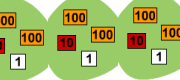Visual Division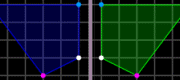Transformation Workshop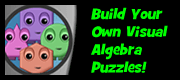DIY Algebra Puzzles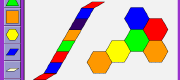Pattern Blocks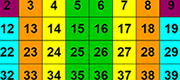Hundreds Chart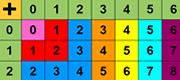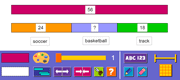Modeling Tool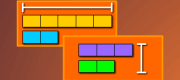Thinking Blocks Ratios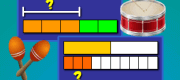Thinking Blocks Fractions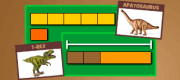Thinking Blocks Multiply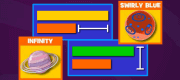Thinking Blocks Junior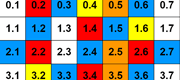Decimal Chart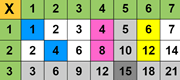Multiplication Chart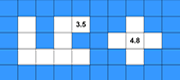Decimal Patterns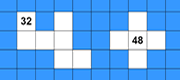Number Patterns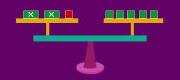Model AlgebraCuisenaire Rods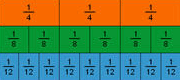Fraction Bars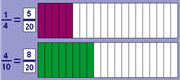Visual FractionsFraction ScaleFraction-Decimal ScaleFraction-Percent ScaleVisual Percent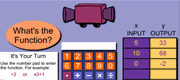Function MachineCircle GraphsSpinners and Probability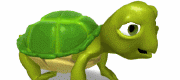Simple ProgrammingArea and Perimeter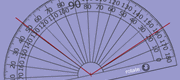Measuring Angles